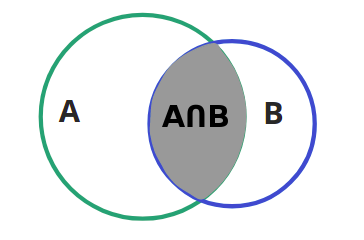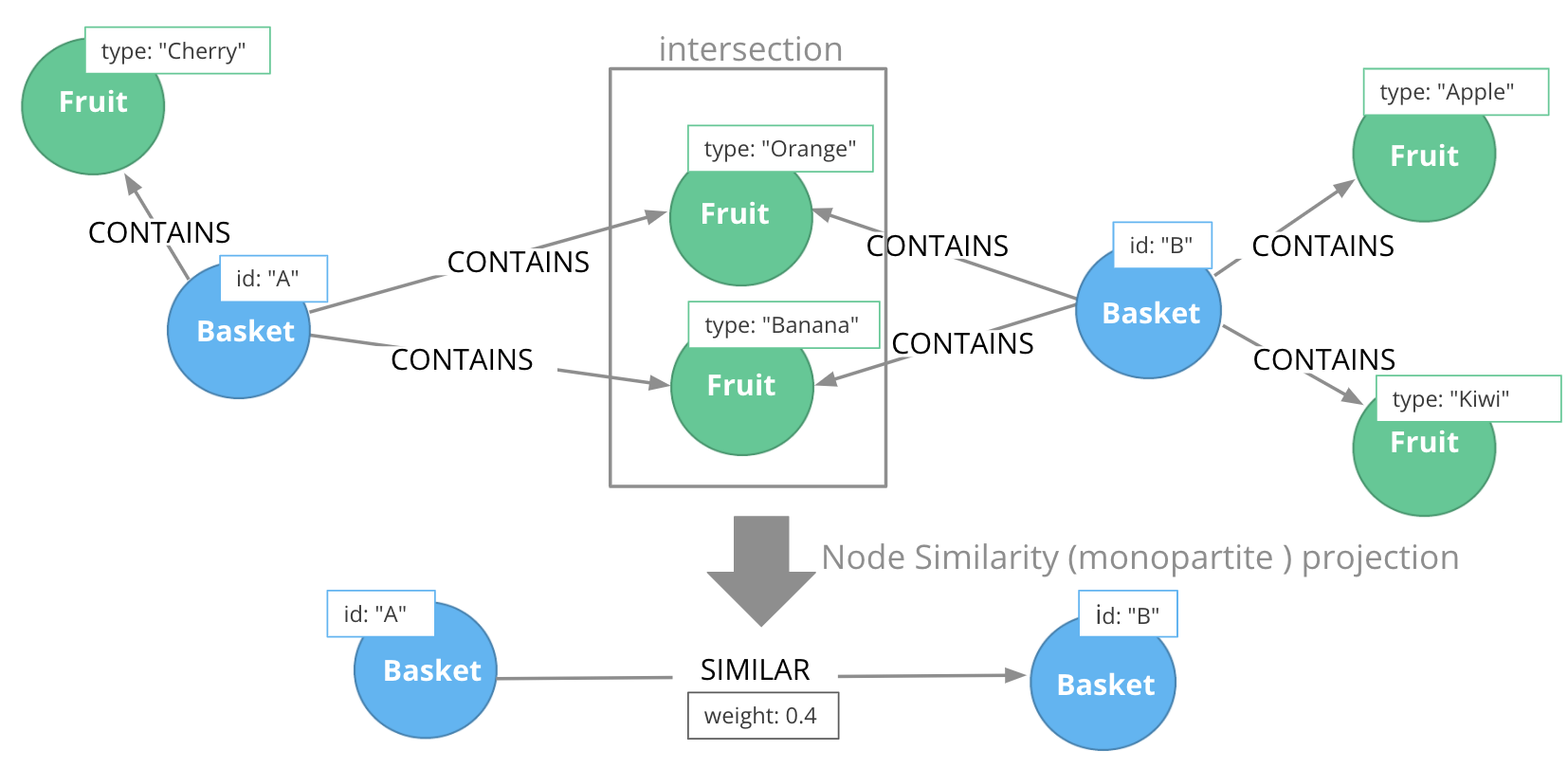# Similarity Algorithms

## Similarity algorithms

Similarity algorithms evaluate how alike nodes are at an individual level based on node properties, neighboring nodes, or relationship properties.

Product supported:

• Node Similarity (Jaccard Index)

Labs implementations:

• Cosine Similarity

• Euclidean Similarity

• Overlap Similarity

• Pearson Similarity

• Approximate Nearest Neighbors

## Node Similarity algorithm

The Node Similarity algorithm computes similarities between pairs of nodes based on the Jaccard Similarity Score. Two nodes are considered similar if they share many of the same neighbors.

The input of this algorithm is usually a bipartite graph containing two disjoint node sets. The Node Similarity algorithm compares all nodes from the first node set based on their relationships to nodes in the second set. The output of the algorithm is a unipartite network between nodes in the first node set. We can think of this process as translating indirect relationships to direct ones. Mathematically, Jaccard Similarity Score is defined as the size of the intersection divided by the size of the union of two sets.### Example: Node Similarity

For example, if Basket A contains {Orange, Banana, Cherry} and Basket B contains {Orange, Banana, Apple, Kiwi} then the Jaccard algorithm counts 2 co-occurrences {Orange, Banana} and divides that count by the number of items in A and B (while not double-counting items), in this case 5 {Orange, Banana, Apple, Cherry, Kiwi}. The resulting Jaccard Similarity Coefficient is 2/5 which is 0.4. A coefficient of 1 indicates that the compared sets are identical.### Why use Node Similarity?

Here is why you use Node Similarity:

• Find recommendations of similar items

• First step of analyzing a bipartite network

• Part of link prediction analysis

## Guided Exercise: Getting Started with Node Similarity algorithm

Follow along with this video to become familiar with Jaccard Similarity in Neo4j NEuler.

## Exercise: Node Similarity

1. In NEuler:

1. Try various algorithm configurations for the Questions dataset.

2. Try other datasets.

2. In Neo4j Browser: :play 4.0-intro-graph-algos-exercises and follow the instructions for Node Similarity.

 Estimated time to complete: 20 minutes

### Question 1

Which Similarity algorithm is fully supported in the Graph Data Science Library?

• Pearson Similarity

• Euclidean Similarity

• Node Similarity (Jaccard Index)

• Overlap Similarity

### Question 2

How is the Jaccard similarity score calculated?

• intersection of two sets divided by the union of sets

• intersection of sets

• union of sets

• union of two sets divided by the intersections of sets

### Question 3

The Node Similarity algorithm calculates a Jaccard Index for each node. What value indicates that compared sets are identical?

• 0

• 1

• 10

• 100

## Summary

In this lesson you gained some experience with the Neo4j supported Node Similarity (Jaccard Index) algorithm.